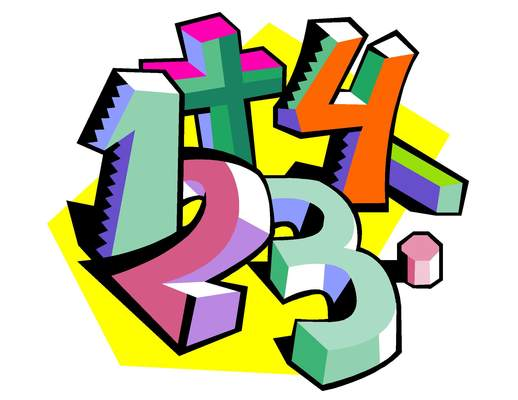3 years ago
kbassett
Save
Edit
Host a game
Live GameLive
Homework
Solo Practice
Practice20 QuestionsShow answers
• Question 1
10 seconds
Q. 4+5=
9
11
10
8
• Question 2
10 seconds
Q. 9+3=
12
11
03
10
• Question 3
10 seconds
Q. 7+4=
11
10
12
13
• Question 4
10 seconds
Q. 8+7=
15
14
13
16
• Question 5
10 seconds
Q. 8+5=
13
15
12
11
• Question 6
10 seconds
Q. 9+6=
15
16
14
17
• Question 7
10 seconds
Q. 8+9=
17
16
18
15
• Question 8
10 seconds
Q. 9+9=
18
17
19
16
• Question 9
10 seconds
Q. 8+8=
16
14
18
15
• Question 10
10 seconds
Q. 3+8=
11
10
12
13
• Question 11
10 seconds
Q. 6+7=
13
12
14
15
• Question 12
10 seconds
Q. 7+9=
16
15
17
18
• Question 13
10 seconds
Q. 4+9=
13
12
14
15
• Question 14
10 seconds
Q. 5+9=
14
13
15
12
• Question 15
10 seconds
Q. 4+8=
12
11
13
14
• Question 16
30 seconds
Q. 8+6=
14
15
13
12
• Question 17
10 seconds
Q. 8+9=
17
16
18
15
• Question 18
10 seconds
Q. 7+7=
14
13
12
15
• Question 19
10 seconds
Q. 4+9=
13
12
14
11
• Question 20
10 seconds
Q.